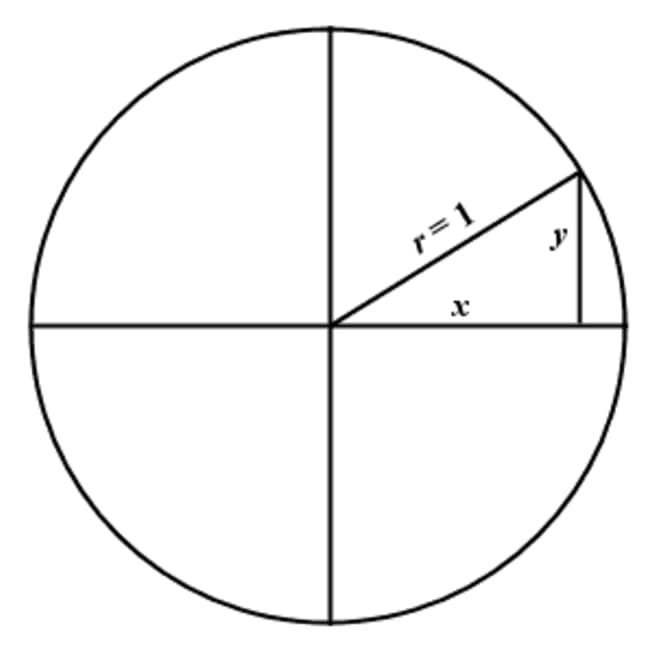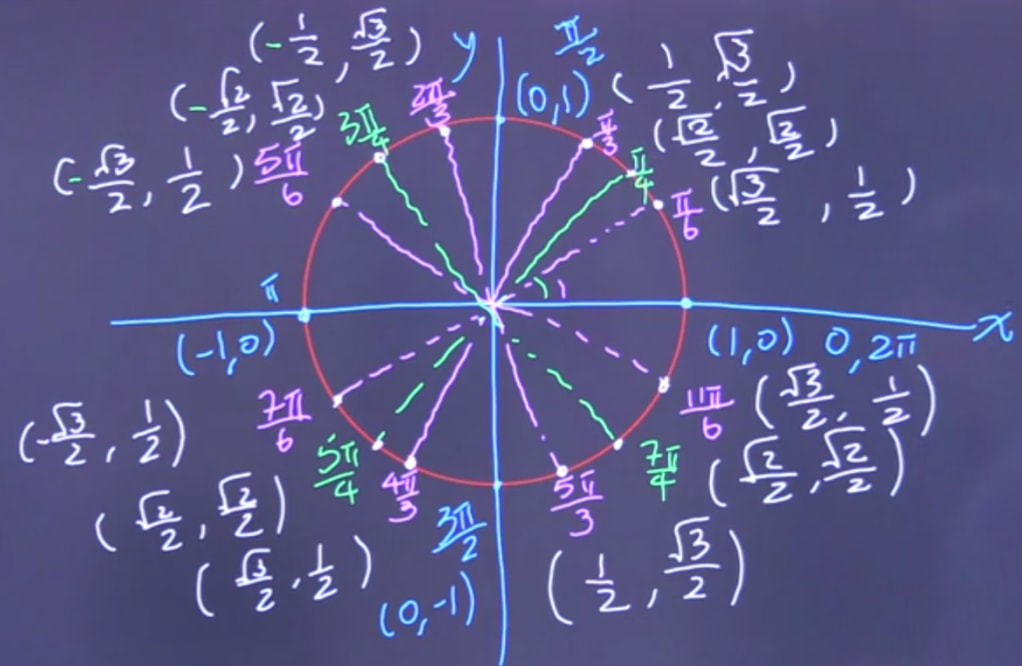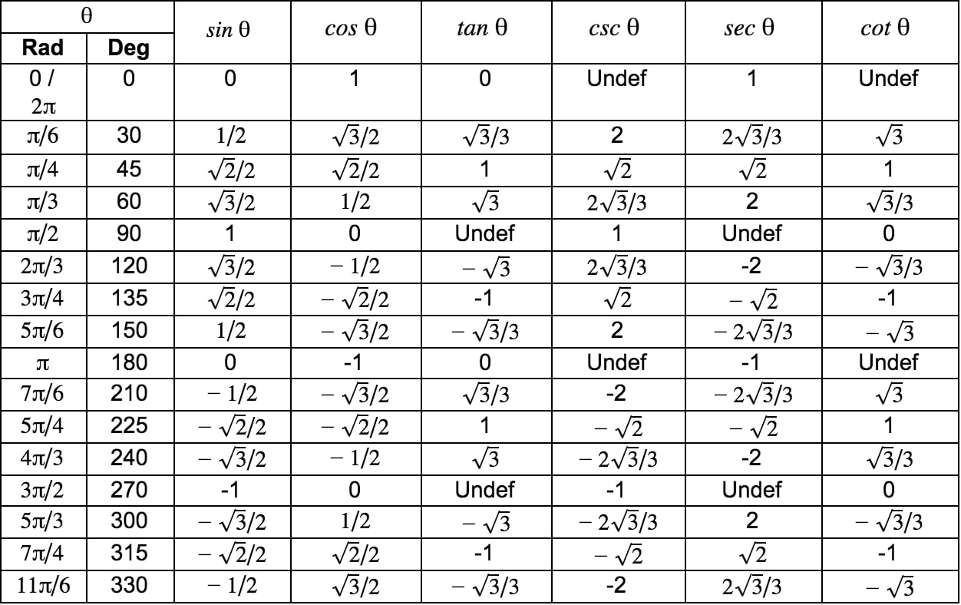# Unit circle

## What is Unit Circle?

In the world of calculus, pre-calculus, and trigonometry, you will often find reference toward and problems regarding "the unit circle." But, oddly, we are rarely ever taught what it is!

In simple terms, the unit circle is a mathematical tool for making the use of angles and trigonometric functions easier. By understanding and memorizing "the unit circle" we are able to breeze through otherwise calculation-heavy problems, and make our lives a whole lot easier.

The unit circle, in it's simplest form, is actually exactly what it sounds like: A circle on the Cartesian Plane with a radius of exactly $1 unit$. Like this blank unit circle below:Blank unit circle with radius of 1

Next, by filling in this unit circle with commonly used angles and evaluating these angles with sine and cosine, we get something a $little$ more complicated:Sine and Cosine evaluated angles of unit circle

Scared? Don't be. This image might seem intimidating, but when we break it down it more coherent parts, patterns start to emerge.

## Unit Circle With All 6 Trig Functions Chart:

Instead of referring to that intimidating image above, let's simplify the unit circle with $\sin \cos \tan \sec \csc$ and $\cot$ in a nice little chart:Chart of simplified unit circle with sin cos tan sec csc and cot

This above unit circle table gives all the unit circle values for all 4 unit circle quadrants. As you can see, listed are the unit circle degrees and unit circle radians. You should know both, but you're most likely to be solving problems in radians. Now, the next natural question is, how can I remember the unit circle?

## How to Memorize the Unit Circle:

Memorizing the unit circle is actually much easier than you'd think, thanks to a couple little tricks:

Trick 1:

Because of the following 4 equations, we only need to memorize the unit circle values for sine and cosine.

$\tan \theta = \frac{\sin \theta}{\cos \theta}, \cot \theta = \frac{\cos \theta}{\sin \theta}, \sec \theta = \frac{1}{\cos \theta}, \csc \theta = \frac{1}{\sin \theta}$

With these 4 equations, we don't even need to memorize the unit circle with tangent!

Trick 2:

By knowing in which quadrants x and y are positive, we only need to memorize the unit circle values for sine and cosine in the first quadrant, as the values only change in their sign. To use this trick, there are a few things we need to understand first:

i) The first important thing to note is what values sine and cosine give us on the unit circle. Because of SOHCAHTOA, we know this:

$\sin \theta$ gives us the Y-coordinate and $\cos \theta$ gives us the X-coordinate

ii) Now looking at each quadrant:

Quadrant 1: X is Positive, Y is Positive

Quadrant 2: X is Negative, Y is Positive

Quadrant 3: X is Negative, Y is Negative

Quadrant 4: X is Positive, Y is Negative

iii) Next, looking at where each quadrant lies:

Quadrant 1: 0 – $\frac{\pi}{2}$

Quadrant 2: $\frac{\pi}{2} - \pi$

Quadrant 3: $\pi$$\frac{3\pi}{2}$

Quadrant 4: $\frac{3\pi}{2} - 2\pi$

iv) The value of sine and cosine will always be "the same" for the same denominator:

$\sin \frac{\pi}{3} = \frac{\sqrt{3}}{2} and \sin \frac{4\pi}{3} = -\frac{\sqrt{3}}{2}$

With these tricks in mind, the process of how to remember the unit circle becomes so much easier!

## How to Use the Unit Circle:

The best way to get comfortable with using the unit circle is to do some unit circle practice.

Example 1:

Find $\sin \frac{4\pi}{3}$

Step 1: Identify The Quadrant

Since we're dealing with sine, which we will eventually have memorized, all we need to do is figure out what quadrant we're in so we know whether our answer will be positive or negative.

Since:

$\frac{3\pi}{2} > \frac{4\pi}{3} > \pi$

We are therefore in the third quadrant. Thus, since sine gives us the y coordinate, and we are in the third quadrant, our answer will be negative!

Step 2: Solve

The next step is simple – using what we've memorized, we can easily solve this problem.

$\sin \frac{4\pi}{3}$ = -$\frac{\sqrt{3}}{2}$

Example 2:

Find $\tan \pi$

Step 1: Identify The Quadrant

Since we're dealing with the unit circle with tan, we will need to use the values we've memorized from sine and cosine, and then solve. First, however, we need to figure out what quadrant we're in so we know whether our answers for sine and cosine will be positive or negative.

Since:

$\frac{3\pi}{2} > \pi > \frac{\pi}{2}$

We are therefore in between the second and third quadrant on the x-axis. Since sine gives us the y coordinate, and we are on the x-axis, our answer will actually be zero! Also, since cosine gives us the x-coordinate, and we are in between the second and third quadrant (where cosine is negative for both), our answer will be negative!

Step 2: Solve

The next step is simple – using what we've memorized, we can easily solve this problem. But in this case, we need one extra step. We must use the equation for tangent discussed earlier in trick 1, assuming we haven't memorized the values for tangent on the unit circle.

$\tan \theta = \frac{\sin \theta}{\cos \theta} = \frac{0}{-1} = 0$

Example 3:

Find $\csc \frac{\pi}{6}$

Step 1: Identify The Quadrant

Since we're dealing with cosecant, it is important to recognize we will need to use sine values to solve using the equation for cosecant discussed earlier in trick 1. First, however, we need to figure out what quadrant we're in so we know whether our answer for sine will be positive or negative.

Since:

$\frac{\pi}{2} > \frac{\pi}{6} > 0$

We are therefore in the first quadrant. Thus, since sine gives us the y coordinate, and we are in the first quadrant, our answer will be positive!

Step 2: Solve

The next step is simple – using what we've memorized, we can easily solve this problem. But in this case again, we need one extra step. We must use the equation for cosecant discussed earlier in trick 1, assuming we haven't memorized the values for cosecant on the unit circle.

$\csc \frac{\pi}{6} = \frac{1}{\sin \frac{\pi}{6}} = \frac{1}{0.5} = 2$

Now that we've done some practice, do some more on your own! In no time at all, you'll be ready for any upcoming unit circle quiz.

### Unit circle

#### Lessons

• Introduction
What is unit circle?

• 1.
Using the unit circle, find the following trigonometric ratios of special angles:
sin 0°
cos 0°
sin 90°
cos 90°
sin 180°
cos 180°
sin 270°
cos 270°
sin 360°
cos 360°